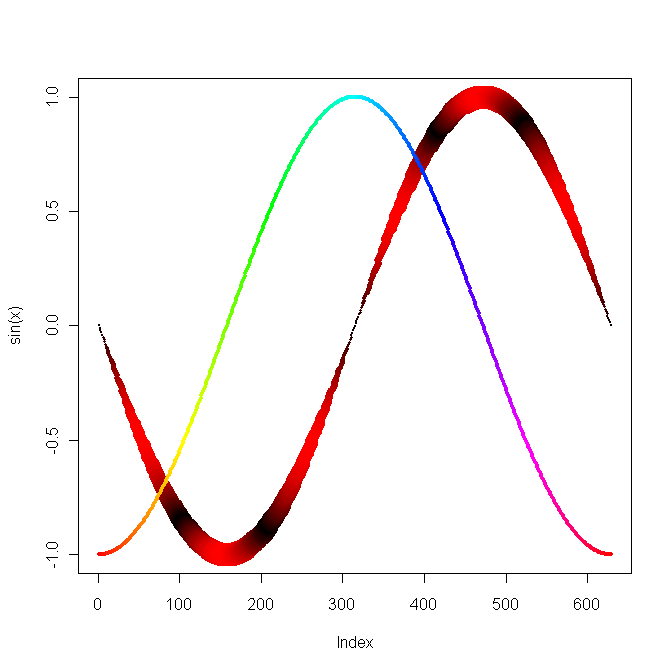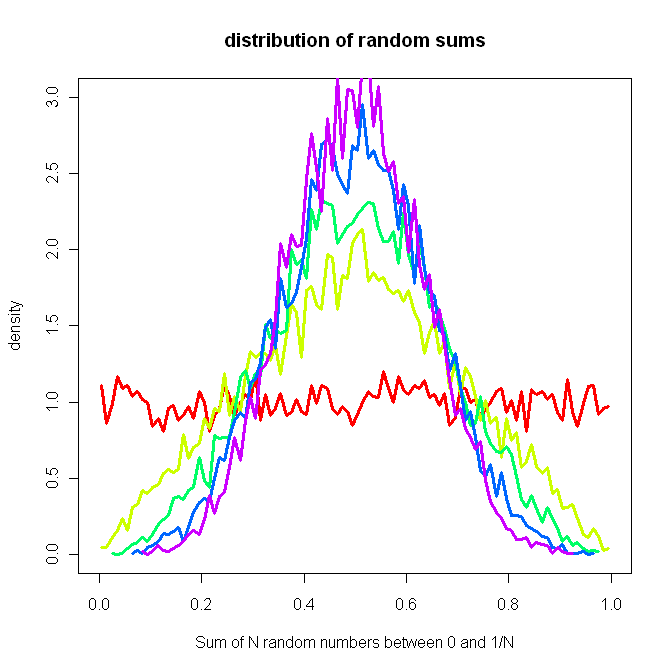## Wednesday, 18 November 2009

### The R Project for Statistical Computing

I've been playing with The R Project for Statistical Computing. I quite like it.

Here's a few of the first things I did, just playing around:`x = seq(-pi, pi, by = 0.01)plot(sin(x), col = rgb(abs(sin(x*3)), 0, 0), cex=.2+3*abs(sin(x)), pch=16)points(cos(x), pch=16, cex=.5, col = rainbow(length(x)))``n = 10000breaks = 100plot_count = 5cols = rainbow(plot_count)plot(x = c(), xlim=c(-0,1), ylim=c(0,3), main = "distribution of random sums", xlab = "Sum of N random numbers between 0 and 1/N", ylab = "density")runifrep = function(n, reps) { tot = 0; for (i in 1:reps) {  tot = tot + runif(n) } tot/reps;}for (i in 1:plot_count) { a = hist(runifrep(n, i), breaks=breaks, plot=0) lines(y = a\$density, x = a\$mids, col = cols[i], pch=3, cex = .1, xlim=c(-4,4), lwd = 3)}`

Note that cex is symbol size, pch is symbol and lwd is line width. http://www.harding.edu/fmccown/R/#misc has a nice list of symbols.